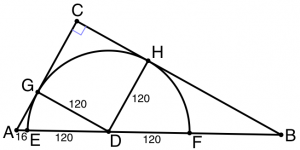## ________________

The students are excited about the Kentucky Derby this weekend, and it shows in their problems.

## ________________### Uneven Odds

Some team members are watching a different race to get in the mood for the Derby, and because of late scratches, there are only four horses running. The odds on the four horses are listed at 5-1, 4-1, 3-1, and 2-1. (Odds of 4-1, for example, mean that a winning ticket bought for \$2 will pay back \$10, providing a net gain of \$8—four times the \$2 bet.) One team member exclaims, “If only we were at the track, we could guarantee that we would make money on this race!”

What is the total amount of money you could bet on this race (possibly different amounts on different horses) so that you would end up with a net gain of exactly \$3 no matter how the race turns out? (Assume that there is a single valid winning horse and ignore any payoffs except for the winner.)

### Stable Selection

A stable has 16 horses and wants to select the three fastest. There’s only room on the practice track to race four horses at a time, and the track has no timer, so the only information from each race is the order in which the horses finish. (Assume for simplicity that each horse runs the track in exactly the same amount of time in each race and that no two horses run the course in exactly the same amount of time.)

What is the smallest number of races the stable can run on the practice track to determine the three fastest horses?

## Solutions to week 85

Peri-area? We want to find whole numbers a, b, and c so that a² + b² = c² and ab/2 = a + b + c. Solving the second equation for c and squaring, we get that c² = a²b²/4 + a² + b² – a²b – ab² + 2ab. But the left hand side is just a² + b² by the first equation, so canceling we have that 0 = a²b²/4 – a²b – ab² + 2ab. Multiplying by 4/ab (which is OK because we know that ab is definitely not zero), we have that 0 = ab – 4a – 4b + 8. Solving for a, we find that a = (4b – 8)/(b – 4) = 4 + 8/(b – 4). Since a is a whole number, we conclude that 8/(b – 4) must be a whole number, or that b – 4 must be a factor of eight. Since the only such factors are 1, 2, 4, and 8, we get that b must be 5, 6, 8, or 12; the corresponding a values are then 12, 8, 6, and 5, so there are really just two different perimetric right triangles: 6,8,10 and 5,12,13.

Hypotenuse Partition.Referring to the diagram, it’s clear that the two smaller right triangles AGD and DHB are similar to the main right triangle R = ACB. Also, segment AD has length 136, so using the Pythagorean Theorem, length AG = 64. By similarity, then, length DB = 136×120/64 = 255. That means the fourth segment FB has length 255 – 120 = 135.

## Recent Weeks

Links to all of the puzzles and solutions are on the Complete Varsity Math page.

Come back next week for answers and more puzzles.

[asciimathsf]